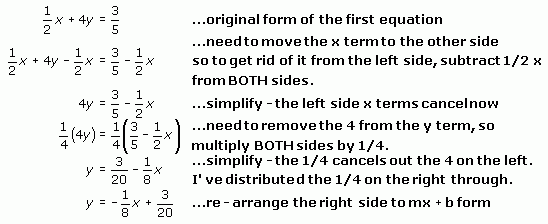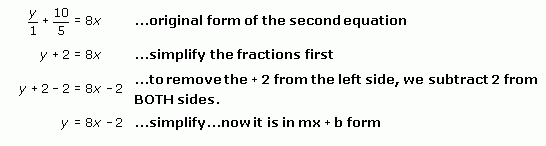SEARCH HOMEMath Central Quandaries & QueriesQuestion from vincent, a student: Can you determine if the two lines are parallel, perpendicular, or neither 1/2x+4y=3/5 y/1 + 10/5= 8x I think there perpendicular but how would you solve the equationsHi Vincent.

Think about the slopes of the lines.  If two lines are parallel, their slopes are the same, right?  The same rise for the same run.

If two lines are perpendicular, what happens to the slope?  It is the negative reciprocal of the other.  For example, a line with slope -3 is perpendicular to a line with slope 1/3.

Can you find the slopes of your lines and compare them?  That will answer your question.

Hint: Change them to y = mx + b form.

Hope this helps,
Stephen La Rocque.

Vincent wrote back:

what I did is
4y=1/2x+3/5

y=1/8x+3/20

but how would you solve for y/1=1/8x+10/5

is the slope the same 1/8 and 8/1?

I see your trouble. When you work with equations, you have to "solve for" a variable. In this case, you want to solve for y. The way you do this is by performing the same operation on both sides of the equation so that you can get y by itself on the left hand side. Let's do this for the first line's equation now. I'll show you all the steps:Now here is how you do this for the second line:You'll want to practice working with equations with just one variable (usually we practice with equations with just x in them) so you know how to do this before working with two or more variables and two or more equations at once. You can find many of these kinds of problems on our web site by typing solve into the search page.

Now the slopes of the two lines are apparent, because they are the numbers (and signs) in front of the x variable when the line is in the y = mx + b form. So the first line's slope is -1/8 and the second line's slope is 8.

The negative reciprocal of 8 is -1/8 so these two lines are parallel.

Stephen La Rocque. >Math Central is supported by the University of Regina and The Pacific Institute for the Mathematical Sciences.